• +91 9971497814
• info@interviewmaterial.com

# Chapter 5 Complex Numbers and Quadratic Equations Ex-5.3 Interview Questions Answers

### Related Subjects

Question 1 : Solve the equation x2 + 3 = 0

x2 + 3 = 0

On comparing it with ax2 + bx + c =0, we have

a =1, b =0, and c =3

So, the discriminant of the given equation will be

D = b2 –4ac =02 – 4 × 1 × 3 = –12

Hence, the required solutions are: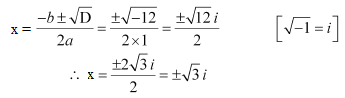Question 2 :

2x2 + x + 1 = 0

2x2 + x +1 = 0

On comparing it with ax2 + bx + c =0, we have

a =2, b =1, and c =1

So, the discriminant of the given equation will be

D = b2 –4ac =12 – 4 × 2 × 1 = 1 – 8 = –7

Hence, the required solutions are: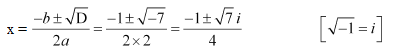Question 3 :

x2 + 3x + 9 = 0

x2 + 3x + 9 = 0

On comparing it with ax2 + bx + c =0, we have

a =1, b =3, and c =9

So, the discriminant of the given equation will be

D = b2 –4ac =32 – 4 × 1 × 9 = 9 – 36 = –27

Hence, the required solutions are: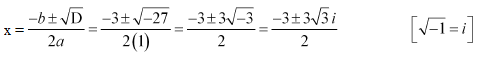Question 4 :

x2 + x – 2 = 0

x2 + –2 = 0

On comparing it with ax2 + bx + c =0, we have

a =–1, b =1, and c =–2

So, the discriminant of the given equation will be

D = b2 –4ac =12 – 4 × (–1) × (–2) = 1 – 8 =–7

Hence, the required solutions are: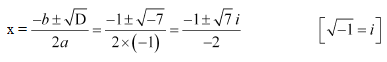Question 5 :

x2 + 3x + 5 = 0

x2 + 3x + 5 = 0

On comparing it with ax2 + bx + c =0, we have

a =1, b =3, and c =5

So, the discriminant of the given equation will be

D = b2 –4ac =32 – 4 × 1 × 5 =9 – 20 = –11

Hence, the required solutions are: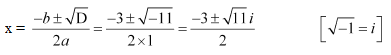Question 6 :

x2 – x + 2 = 0

x2 – x + 2 = 0

On comparing it with ax2 + bx + c =0, we have

a =1, b =–1, and c =2

So, the discriminant of the given equation is

D = b2 –4ac =(–1)2 – 4 × 1 × 2 = 1 – 8 = –7

Hence, the required solutions are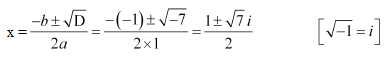Question 7 :

√2x2 + x + √2 = 0

√2x2 + x +√2 = 0

On comparing it with ax2 + bx + c =0, we have

a =√2, b =1, and c =√2

So, the discriminant of the given equation is

D = b2 –4ac =(1)2 – 4 × √2 × √2 = 1 – 8 = –7

Hence, the required solutions are: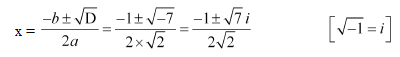Question 8 : √3x2 – √2x + 3√3 = 0

√3x2 –√2x +3√3 = 0

On comparing it with ax2 + bx + c =0, we have

a =√3, b =-√2, and c =3√3

So, the discriminant of the given equation is

D = b2 –4ac =(-√2)2 – 4 × √3 × 3√3 = 2 – 36 =–34

Hence, the required solutions are: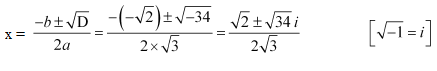Question 9 : x2 + x + 1/√2 = 0

x2 + x + 1/√2 = 0

It can be rewritten as,

√2x2 +√2x +1 = 0

On comparing it with ax2 + bx + c =0, we have

a =√2, b =√2, and c =1

So, the discriminant of the given equation is

D = b2 –4ac =(√2)2 – 4 × √2 × 1 = 2 – 4√2 =2(1 – 2√2)

Hence, the required solutions are: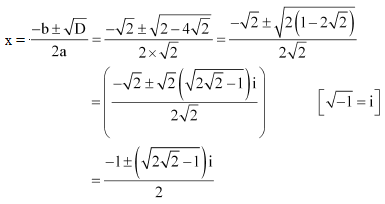Question 10 : x2 + x/√2 + 1 = 0

x2 + x/√2 + 1 = 0

It can be rewritten as,

√2x2 + x +√2 = 0

On comparing it with ax2 + bx + c =0, we have

a =√2, b =1, and c =√2

So, the discriminant of the given equation is

D = b2 –4ac =(1)2 – 4 × √2 × √2 = 1 – 8 = -7

Hence, the required solutions are: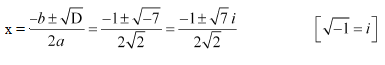Todays Deals### Chapter 5 Complex Numbers and Quadratic Equations Ex-5.3 Contributorskrishan

Name:
Email:

# Latest News# 9000 interview questions in different categories